# Lab 8 - Introduction to Mixture Models

## Structural Equation Modeling - Instructor: Karen Nylund-Gibson

### May 22, 2020

University of California, Santa Barbara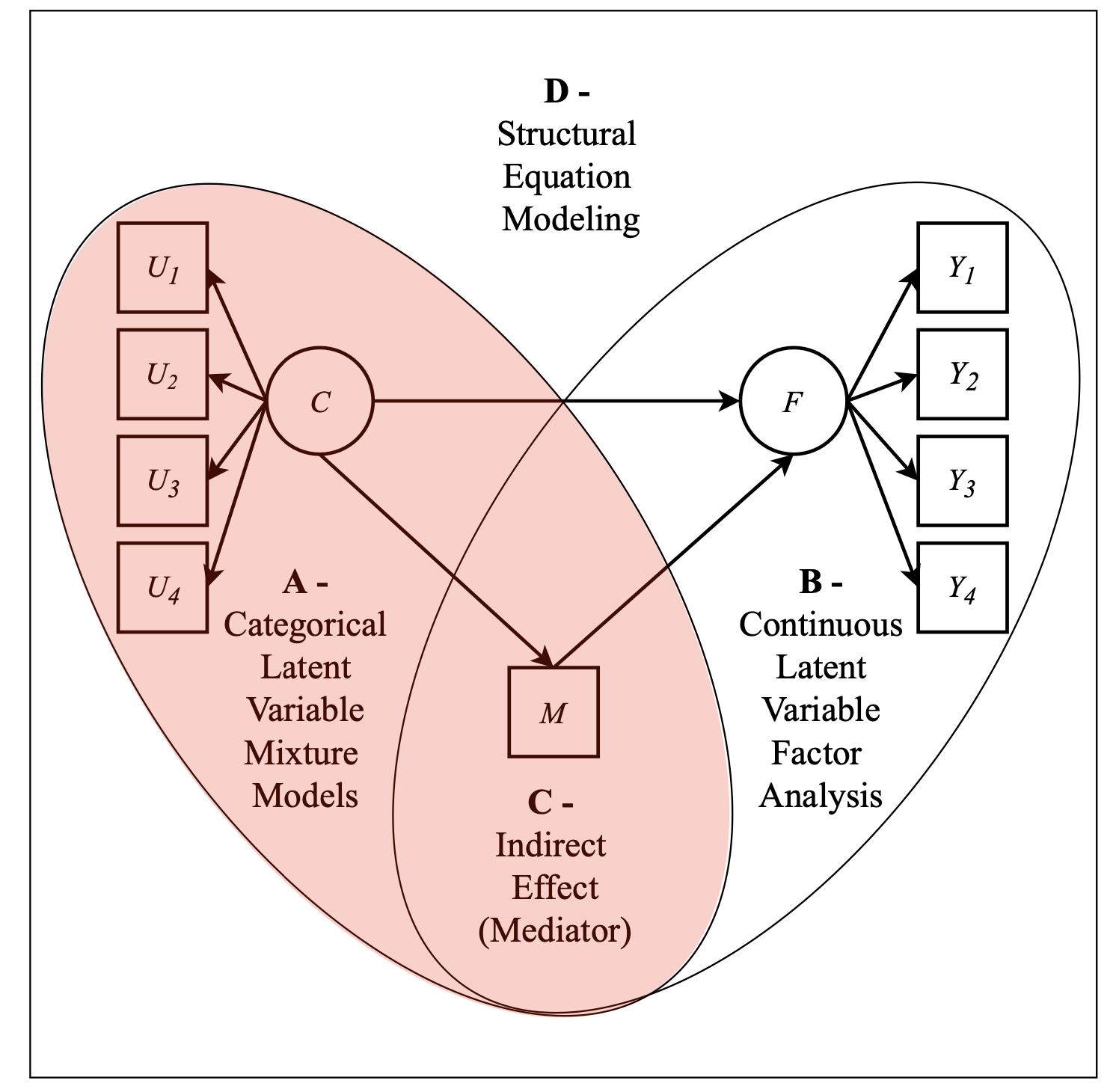Figure. Picture has been adapted from study by Múthen, 2006.

# Lab preparation

## Creating a version-controlled R-Project with Github

On the Github repository webpage:

1. fork your own branch of the lab repository
2. copy the repository web URL address from the clone or download menu

Within R-Studio:

1. click “NEW PROJECT”
2. choose option Version Control
3. choose option Git
4. paste the repository web URL path copied from the clone or download menu on Github page
5. choose location of the R-Project

## Data source:

1. The first example utilizes a dataset on undergraduate Cheating available from the poLCA package (Dayton, 1998): $$\color{blue}{\text{See documentation here}}$$

2. The second examples utilizes the public-use dataset, The Longitudinal Survey of American Youth (LSAY): $$\color{blue}{\text{See documentation here}}$$

3. The third examples utilizes the Kindergarten Student Entrance Profile (KSEP) (Quirk et al., 2011): $$\color{blue}{\text{See documentation here}}$$

library(tidyverse)
library(haven)
library(glue)
library(MplusAutomation)
library(rhdf5)
library(here)
library(janitor)
library(gt)
library(semPlot)
library(reshape2)
library(cowplot)
library(filesstrings)
library(poLCA)

# Enumerate and plot mixtures

Compare k-class models 1 through 6

## Example 1: Undergraduate Cheating behavior

“Dichotomous self-report responses by 319 undergraduates to four questions about cheating behavior” (poLCA, 2016).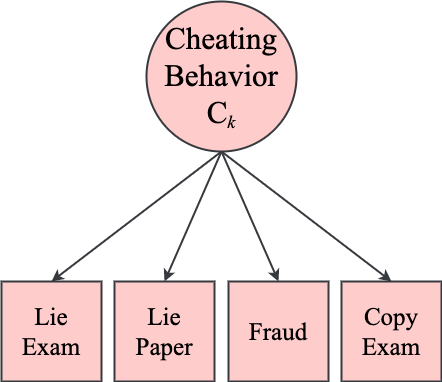LCA indicators1
Name Label Values
LieExam lied to avoid taking an exam 0 = No, 1 = Yes
LiePaper lied to avoid handing a term paper in on time 0 = No, 1 = Yes
Fraud purchased a term paper to hand in as their own or had obtained a copy of an exam prior to taking the exam 0 = No, 1 = Yes
CopyExam copied answers during an exam from someone sitting near to them 0 = No, 1 = Yes

Prepare data

data(cheating)

cheating <- cheating %>% clean_names()

df_cheat <-  cheating %>%                                  #
dplyr::select(1:4) %>%                                   #
dplyr::mutate_all(funs(.-1))                             #

Run a quick LCA using createMixtures

createMixtures(classes = 1:4, filename_stem = "cheat",                                       #
rdata = df_cheat,                                                          #
ANALYSIS = "processors = 10; starts 500 100;",                                #
VARIABLE = "CATEGORICAL = lieexam-copyexam; USEVAR = lieexam-copyexam;",      #
PLOT = "type = plot3; series = lieexam-copyexam(*);")                     #

files <- data.frame(names = list.files(path = here(), full.names = FALSE))

f_cheat <- files %>% dplyr::filter(., grepl('cheat', names))

for (i in 1:length(f_cheat)) { file.move(glue(here("{f_cheat[,i]}")), here("enum_mplus")) }
runModels(here("enum_mplus"), filefilter = "cheat")

View model fit statistics with mixtureSummaryTable()

output_cheat <- readModels(here("enum_mplus"), filefilter = "cheat", quiet = TRUE)

gt(mixtureSummaryTable(output_cheat)) %>%                                                         #
title = "Fit Indices") %>%                                                                    #
tab_options(                                                                                    #
table.width = pct(80)) %>%                                                                    #
tab_footnote(                                                                                   #
footnote = "Undergraduate Cheating Behavior",                                                 #
location = cells_title())                                                                     #
Fit Indices1
Title Classes AIC BIC aBIC Entropy T11_VLMR_PValue T11_LMR_PValue BLRT_PValue min_N max_N min_prob max_prob
1 classes 1 942.876 957.937 945.250 NA NA NA NA 319 319 1.000 1.000
2 classes 2 898.054 931.941 903.395 0.737 0.0000 0.0000 0.0000 54 265 0.818 0.955
3 classes 3 900.471 953.184 908.779 0.840 0.1656 0.1784 0.1395 12 283 0.550 0.982
4 classes 4 910.290 981.829 921.564 0.866 0.6868 0.6882 1.0000 6 290 0.390 0.992

Extract and prepare plot data

# extract posterior probabilities
plot1 <- as.data.frame(output_cheat[["cheat_4_class.out"]][["gh5"]][["means_and_variances_data"]][["estimated_probs"]][["values"]][seq(2,
8, 2), ])  #seq('from','to','by')

# extract class size proportions
c_size <- as.data.frame(output_cheat[["cheat_4_class.out"]][["class_counts"]][["modelEstimated"]][["proportion"]])

colnames(c_size) <- paste0("cs")

c_size <- c_size %>% mutate(cs = round(cs * 100, 2))

# rename columns (classes) and 'Var' (indicator names)
colnames(plot1) <- paste0("C", 1:4, glue(" ({c_size[1:4,]}%)"))
plot1 <- cbind(Var = paste0("U", 1:4), plot1)

# choose the order of indicators by changing to ordered factor
plot1$Var <- fct_inorder(plot1$Var)

# change dateframe from wide to long format
pd_long1 <- melt(plot1, id.vars = "Var")

Plot 4-class latent class posterior probability plot

ggplot(pd_long1, aes(Var, value, shape = variable,                                              #
colour = variable, lty = variable)) +                                       #
geom_point(size = 4) + geom_line(aes(as.integer(Var))) +                                      #
scale_x_discrete(labels = c("Lie Exam", "Lie Paper", "Fraud", "Copy Exam")) +                 #
scale_y_continuous("Probability") +                                                           #
scale_colour_viridis_d(end = .7) +                                                            #
theme_cowplot() + labs(x=" ") +                                                               #
theme(text=element_text(family="Times New Roman", size=12),                                   #
legend.key.width = unit(.5, "line"),                                                    #
legend.text = element_text(family="Times New Roman", size=12),                          #
legend.title = element_blank(),                                                         #
legend.position = "top")                                                                #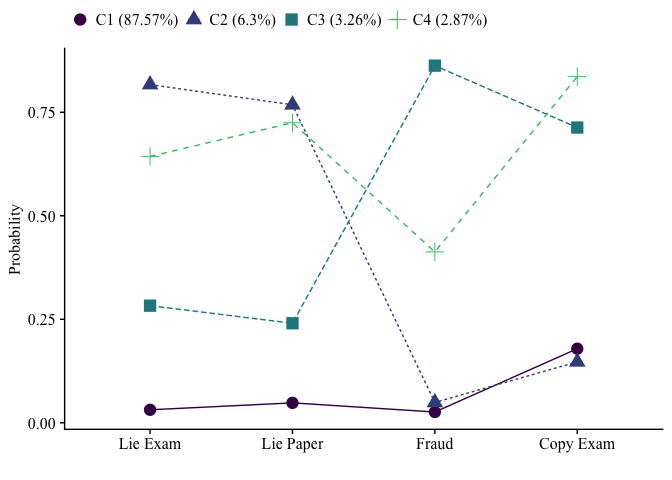save figure

ggsave(here("figures", "C4_Cheat_LCA_Plot.png"), dpi = "retina", height = 5, width = 7,
units = "in")

## Example 2: Longitudinal Study of American Youth, Science Attitudes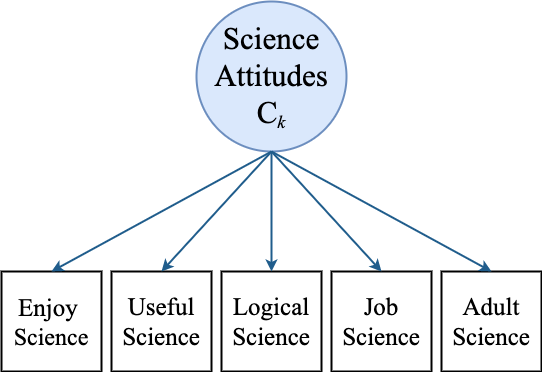lsay_data <- read_csv(here("data", "lca_lsay_sci.csv"), na = c("9999", "9999.00")) %>%               #
clean_names() %>%                                                                                  #
dplyr::select(1:5, Enjoy = ab39m, Useful = ab39t,                                                  #
Logical = ab39u, Job = ab39w, Adult = ab39x)                                    #

View LCA indicators

LCA Indicators1
Name Label Values
Enjoy I enjoy science 0 = Disagree, 1 = Agree
Useful Science useful in everday problems 0 = Disagree, 1 = Agree
Logical Science helps logical thinkng 0 = Disagree, 1 = Agree
Job Need science for a good job 0 = Disagree, 1 = Agree
Adult Will use science often as an adult 0 = Disagree, 1 = Agree

1 Longitudinal Study of American Youth

Run enumeration using mplusObject method

lca_k1_6  <- lapply(1:6, function(k) {
lca_enum  <- mplusObject(

TITLE = glue("Class {k}"),

VARIABLE = glue(
classes = c({k}); "),

ANALYSIS =
"estimator = mlr;
type = mixture;
stseed = 5212020;
starts = 200 100;
processors = 10;",

OUTPUT = "sampstat residual tech11 tech14;",

PLOT =
"type = plot3;

usevariables = colnames(lsay_data),
rdata = lsay_data)

lca_enum_fit <- mplusModeler(lca_enum,
dataout=glue(here("enum_mplus", "c_lca_lsay_Lab8.dat")),
modelout=glue(here("enum_mplus", "c{k}_lca_lsay_Lab8.inp")) ,
check=TRUE, run = TRUE, hashfilename = FALSE)
})

Compare model fit for series of enumerated models

all_output <- readModels(here("enum_mplus"), filefilter = "lsay", quiet = TRUE)

enum_summary <- LatexSummaryTable(all_output,                                             #
keepCols=c("Title", "LL", "BIC", "aBIC",                                  #
"BLRT_PValue", "T11_VLMR_PValue"),                             #
sortBy = "Title")                                                         #

gt(enum_summary)  %>%                                                                     #
title = "Fit Indices" ) %>%                                                           #
tab_options(                                                                            #
table.width = pct(80)) %>%                                                            #
tab_footnote(                                                                           #
footnote = "Longitudinal Study of American Youth",                                    #
location = cells_title())                                                             #
Fit Indices1
Title LL BIC aBIC BLRT_PValue T11_VLMR_PValue
Class 1 -10250.604 20541.34 20525.45 NA NA
Class 2 -8785.317 17658.92 17623.97 0 0.0000
Class 3 -8693.569 17523.59 17469.57 0 0.0000
Class 4 -8664.090 17512.79 17439.71 0 0.0000
Class 5 -8662.386 17557.54 17465.40 1 0.6734
Class 6 -8661.541 17604.01 17492.80 1 0.8384

1 Longitudinal Study of American Youth

Compare probability plots for $$K = 1:6$$ class solutions

model_results <- data.frame()

for (i in 1:length(all_output)) {

temp <- all_output[[i]]$parameters$unstandardized %>%                                            #
mutate(model = paste(i, "-Class Model"))                                                       #

model_results <- rbind(model_results, temp)
}

rm(temp)

model_results <- model_results %>%                                                                 #
dplyr::select(est, model, LatentClass, param) %>%                                                #
mutate(prob = (1 / (1 + exp(est)))) %>%                                                          #
mutate(param = as.factor(str_to_lower(str_sub(param, end = -3)))) %>%                            #
dplyr::select(-est)                                                                              #

ggplot(model_results, aes(x = param, y = prob,                                                     #
color = LatentClass, shape = LatentClass,                                               #
group = LatentClass, lty = LatentClass)) +                                              #
geom_point() + geom_line() +                                                                     #
scale_colour_viridis_d(end = .8, direction = -1) +                                               #
facet_wrap(~ model, ncol = 2) +                                                                  #
labs(title = "LCA Posterior Probability Plot",                                                   #
x= "Science attitudes", y = "Probability") +                                                #
theme_minimal()                                                                                  #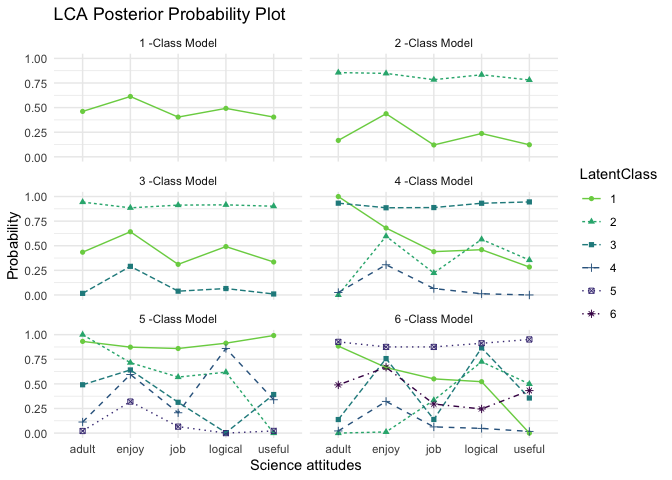ggsave(here("figures", "Enum_LCA_facet_plot.png"), dpi = 300, height = 4, width = 6,
units = "in")

## Example 3 - Kindergarten Student Entrance Profile (KSEP)

ksep <- read_csv(here("data", "KSEP_sub_L6.18.csv"))
LCA Indicators1
Name Label Values
seek_hlp Seeks adult help when appropriate 0 = Not Mastered, 1 = Mastered
cooperat Engages in cooperative play activities with peers 0 = Not Mastered, 1 = Mastered
imp_cntr Exhibits impulse control and self-regulation 0 = Not Mastered, 1 = Mastered
repeats Stays with or repeats a task 0 = Not Mastered, 1 = Mastered
separate Separates appropriately from caregiver most days 0 = Not Mastered, 1 = Mastered
new_activ Is enthusiastic and curious in approaching new activities 0 = Not Mastered, 1 = Mastered
folw_rul Follows rules when participating in routine activities 0 = Not Mastered, 1 = Mastered
name Recognizes own name 0 = Not Mastered, 1 = Mastered
writes Writes own name 0 = Not Mastered, 1 = Mastered
express Demonstrates expressive abilities 0 = Not Mastered, 1 = Mastered
quantity Understands that numbers represent quantity 0 = Not Mastered, 1 = Mastered
colors Recognizes Colors 0 = Not Mastered, 1 = Mastered
shapes Recognizes primary shapes 0 = Not Mastered, 1 = Mastered

1 Kindergarten Student Entrance Profile

Enumeration: Compare k-class models 1-6

lca_k1_6  <- lapply(1:6, function(k) {
lca_enum  <- mplusObject(

TITLE = glue("Class {k}"),

VARIABLE = glue(
"categorical = seek_hlp-shapes;
usevar = seek_hlp-shapes;
classes = c({k}); "),

ANALYSIS =
"estimator = mlr;
type = mixture;
stseed = 5212020;
starts = 200 100;
processors = 10;",

OUTPUT = "sampstat residual tech11 tech14;",

PLOT =
"type = plot3;
series = seek_hlp-shapes(*);",

usevariables = colnames(ksep),
rdata = ksep)

lca_enum_fit <- mplusModeler(lca_enum,
dataout=glue(here("enum_ksep", "c_lca_ksep_Lab8.dat")),
modelout=glue(here("enum_ksep", "c{k}_lca_ksep_Lab8.inp")) ,
check=TRUE, run = TRUE, hashfilename = FALSE)
})

Compare model fit for series of enumerated models

all_output <- readModels(here("enum_ksep"), quiet = TRUE)

enum_summary <- LatexSummaryTable(all_output,                                              #
keepCols=c("Title", "LL", "BIC", "aBIC",                                   #
"BLRT_PValue", "T11_VLMR_PValue"),                              #
sortBy = "Title")                                                          #

gt(enum_summary) %>%                                                                       #
title = "Fit Indices") %>%                                                             #
tab_options(                                                                             #
table.width = pct(80)) %>%                                                             #
tab_footnote(                                                                            #
footnote = "Kindergarten Student Entrance Profile",                                    #
location = cells_title())                                                              # 
Fit Indices1
Title LL BIC aBIC BLRT_PValue T11_VLMR_PValue
Class 1 -11844.461 23783.62 23742.32 NA NA
Class 2 -9712.793 19622.26 19536.49 0 0.0000
Class 3 -9372.043 19042.74 18912.49 0 0.0000
Class 4 -9215.475 18831.58 18656.86 0 0.1240
Class 5 -9137.866 18778.34 18559.15 0 0.0722
Class 6 -9075.565 18755.71 18492.05 0 0.0394

1 Kindergarten Student Entrance Profile

Compare probability plots for $$K = 1:6$$ class solutions

model_results <- data.frame()

for (i in 1:length(all_output)) {

temp <- all_output[[i]]$parameters$unstandardized %>%                                       #
mutate(model = paste(i, "-Class Model"))                                                  #

model_results <- rbind(model_results, temp)
}

rm(temp)

model_results <- model_results %>%                                                                         #
dplyr::select(est, model, LatentClass, param) %>%                                                        #
mutate(prob = (1 / (1 + exp(est)))) %>%                                                                  #
mutate(param = as.factor(str_to_lower(str_sub(param, end = -3)))) %>%                                    #
dplyr::select(-est)                                                                                      #

model_results$param <- fct_inorder(model_results$param)

ggplot(model_results, aes(x = param, y = prob,                                                             #
color = LatentClass, shape = LatentClass,                                                       #
group = LatentClass, lty = LatentClass)) +                                                      #
geom_point() + geom_line() +                                                                             #
scale_colour_viridis_d() +                                                                               #
facet_wrap(~ model, ncol = 2) +                                                                          #
labs(title = "Kindergarten Student Entrance Profile (KSEP)",                                             #
x= " ", y = "Probability") +                                                                        #
scale_x_discrete(labels = c("Seeks help", "Cooperative", "Impulse control","Repeats", "Separates",       #
"New activities", "Follows rules", "Name", "Writes", "Expressive", "Quantity",        #
"Colors", "Shapes")) +                                                                #
theme_minimal() + theme(panel.grid.major.y = element_blank(),                                            #
axis.text.x = element_text(angle = -45, hjust = -.1))                            #